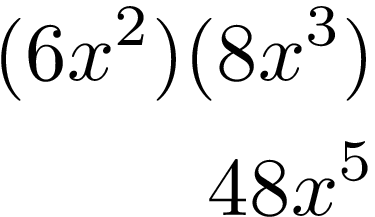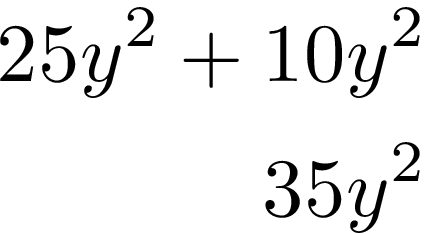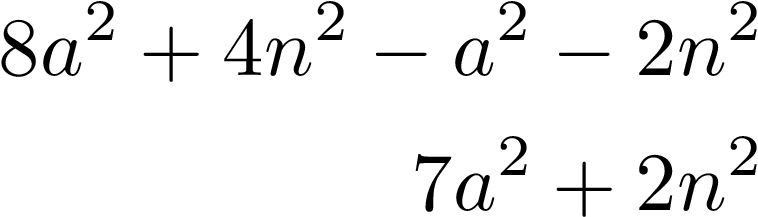# Polynomials

Polynomial is an expression that has more than one algebraic term. Below is an example,Of course, there is much more to polynomials then this simple definition. This post will explain how to deal with polynomials in various situations.

To add and subtract polynomials you must combine like terms. Below is an exampleAll we did was combine the terms that had the y^2 in them. This, of course, applies to subtraction as well.In the example above, the terms with “a” in them are combined and the terms with “n” in then are combined.

Exponents

When dealing with exponents when multiplication is involved you add the exponents together.Notice how like terms were dealt with separately.

Since we add exponents during multiplication we subtract them during division.The 2 in the numerator and denominator cancel out and  7 – 5 = 2.

When parentheses are involved it is a little more complicated. For example, when the exponent is negative you multiply the exponents belowFor negative exponents, you fill the numerator and denominator around and make the negative exponent positive.There are other concepts involving polynomials not covered here. Examples include long division with polynomials and synthetic division. These are fascinating concepts however in the books I have consulted, once these concepts are taught they are never used again in future chapters. Therefore, perhaps they are simply interesting but not commonly used in practice.

Conclusion

Understanding polynomials is critical to future success in algebra. As concepts become more advanced, it will seem as if you are always trying to simplify terms using concepts learned in relation to polynomials.